﻿

### On the domain of a Fleming--Viot-type operator on an$L^p$-space with invariant measure

#### Abstract

We characterize the domain of a Fleming-Viot type operator of the form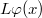$L\varphi(x)$:=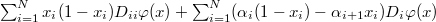$\sum_{i=1}^Nx_i(1-x_i)D_{ii}\varphi(x)+\sum_{i=1}^N(\alpha_i(1-x_i)-\alpha_{i+1}x_i)D_i\varphi(x)$ on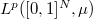$L^p([0,1]^N,\mu)$ for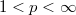$1, where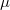$\mu$ is the corresponding invariant measure. Our approach relies on the characterization of the domain of the one-dimensional Fleming-Viot operator and the Dore-Venni operator sum method.

DOI Code: 10.1285/i15900932v31n1p139

Keywords: Fleming--Viot process; degenerate elliptic problems; analytic $C_0$-semigroups

Full Text: PDF